﻿ 基于一种卷积神经式类网络的实时人脸识别方法研究 Study on Convolutional Neural Networks Cascade for Real-time Face Recognition Methods

Computer Science and Application
Vol. 10  No. 01 ( 2020 ), Article ID: 33792 , 10 pages
10.12677/CSA.2020.101002

Study on Convolutional Neural Networks Cascade for Real-time Face Recognition Methods

Jianliang Xu, Mingan Zhou, Jianhui Mao, Kunli Fang

Quzhou College of Technology, Quzhou Zhejiang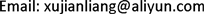Received: Dec. 10th, 2019; accepted: Dec. 27th, 2019; published: Jan. 3rd, 2019ABSTRACT

Facial recognition technology has received extensive attention and massively developed in recent years. Facial recognizing with a traditional feature extractor, such as Local Binary Pattern (LBP), leads to a strong demand for massive training data. The size of the training model is easily affected by the amount of data, which prolongs the computing time of the face recognition system and affects its efficiency. A three-stage tandem face detector and a face recognition method based on the Nearest Feature Line (NFL) are proposed. Feature maps (FM) generated by the Convolutional Neural Network (CNN) is used as the input data for the tandem classifier (Adaboost). Through the analysis of experimental results, the proposed method can build a fast face detector with fewer weak classifiers. In face recognition, a recent feature space transformation is proposed. This method uses point-to-point, point-to-line, and point-to-surface vectors as the basis for covariance calculations. The matrix has a better feature space, that is, this feature space is more general and representative than the feature space obtained from the traditional point-to-point feature space. The method was applied in the actual detection, and its adaptability and accuracy are better.

Keywords:Face Detection, Face Recognition, Neural Network, Adaboost Classifier1. 前言

2. 两阶段串联式人脸检测器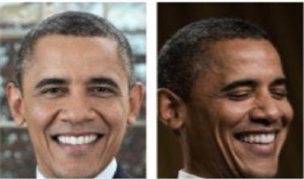(a) 头部姿势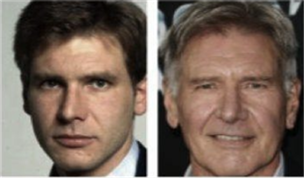(b) 年龄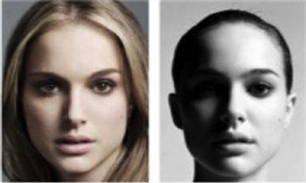(c) 光照(d) 遮挡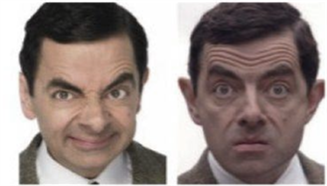(e) 表情

Figure 1. Natural facial image of typical interference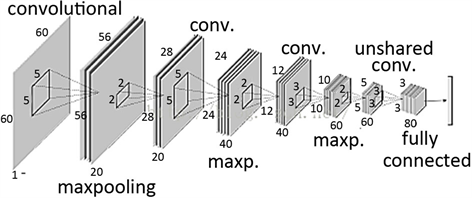Figure 2. Process of convolutional neural networks

1) 人脸检测。人脸检测器用于寻找图像中人脸的位置，如果有人脸，就返回包含每张人脸的边界框的坐标。如图3(a)所示。

2) 人脸对齐。人脸对齐的目标是使用一组位于图像中固定位置的参考点来缩放和裁剪人脸图像。这个过程通常需要使用一个特征点检测器来寻找一组人脸特征点，在简单的2维对齐情况中，即为寻找最适合参考点的最佳仿射变换。图3(b)和图3(c)展示了两张使用了同一组参考点对齐后的人脸图像。更复杂的3D对齐算法还能实现人脸正面化，即将人脸的姿势调整到正面向前。

3) 人脸表征。在人脸表征阶段，人脸图像的像素值会被转换成紧凑且可判别的特征向量，这也被称为模板(template)。理想情况下，同一个主体的所有人脸都应该映像到相似的特征向量。

4) 人脸匹配。在人脸匹配构建模块中，两个模板会进行比较，从而得到一个相似度分数，该分数给出了两者属于同一个主体的可能性。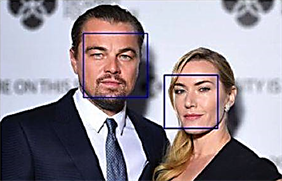(a) 人脸检测器找到的边界框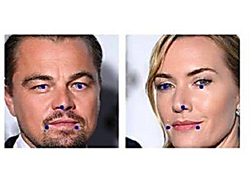(b)和(c)对齐后的人脸和参考点

Figure 3. Face recognition and alignment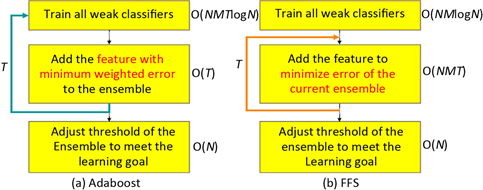Figure 4. The comparison of Adaboost and FFS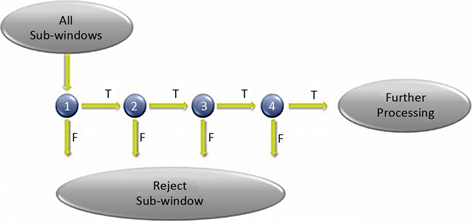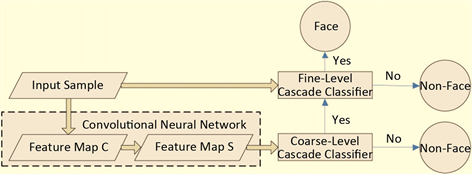Figure 6. System architecture

3. 三阶段人脸识别方法

3.1. 三阶段人脸识别架构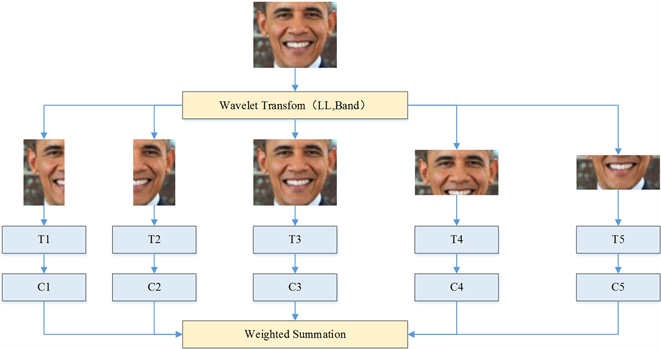Figure 7. Three stage face recognition framework proposed

3.2. 最近特征空间转换法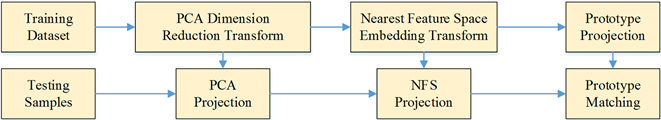Figure 8. Flow chart of nearest feature line transformation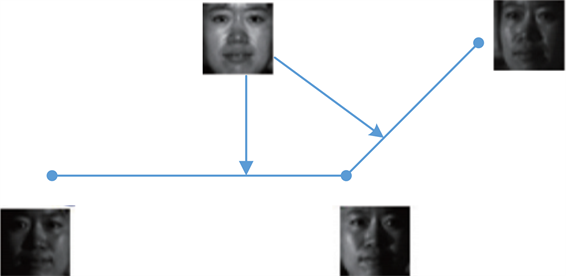Figure 9. Strategy nearest feature line

$\left\{\begin{array}{l}{F}_{1}={\sum _{i}\left[\sum \left({y}_{i}-{f}^{p}\left({y}_{i}\right)\right){w}^{p}\left({y}_{i}\right)\right]}^{2}\\ {F}_{2}=\sum _{i}\sum {\left({y}_{i}-{f}^{p}\left({y}_{i}\right)\right)}^{2}{w}^{p}\left({y}_{i}\right)\end{array}$ (1)

${f}_{m,n}^{2}\left({y}_{i}\right)={y}_{m}+{t}_{m,n}\left({y}_{n}-{y}_{m}\right)$ (2)

${y}_{i}-{f}_{m,n}^{\left(2\right)}\left({y}_{i}\right)={y}_{i}-{t}_{m,n}{y}_{m}+{t}_{m,n}{y}_{n}$ (3)

$1-{t}_{m,n}={t}_{m,n}$ ，若从 ${C}_{N-1}^{2}$ 条线中挑选出K个最近特征线则目标函数(1)可以表示如下：

$\sum _{i}{\left(\sum _{m\ne n}\left({y}_{i}-{f}_{m,n}^{2}\left({y}_{i}\right)\right){w}_{m,n}^{\left(2\right)}\left({y}_{i}\right)\right)}^{2}={W}^{\text{T}}XL{X}^{\text{T}}W$ (4)

${w}_{m,n}^{\left(2\right)}\left({y}_{i}\right)=\left\{\begin{array}{l}1\text{ }\text{ }\text{ }\text{\hspace{0.17em}}\text{\hspace{0.17em}}\text{\hspace{0.17em}}m\ne n\\ 0\text{ }\text{ }\text{\hspace{0.17em}}\text{ }\text{otherwise}\end{array}$ (5)

${M}_{i,n}=\left\{\begin{array}{l}\frac{1}{K}\sum _{m}{t}_{m,n}\text{ }\text{ }\text{ }\text{ }\text{\hspace{0.17em}}\text{\hspace{0.17em}}m\ne n\ne i\\ 0\text{ }\text{ }\text{ }\text{ }\text{ }\text{ }\text{ }\text{ }\text{ }\text{\hspace{0.17em}}\text{\hspace{0.17em}}\text{\hspace{0.17em}}\text{\hspace{0.17em}}\text{\hspace{0.17em}}\text{\hspace{0.17em}}\text{\hspace{0.17em}}\text{\hspace{0.17em}}\text{\hspace{0.17em}}\text{\hspace{0.17em}}\text{ }\text{otherwise}\end{array}$ (6)

$\sum _{i}\sum _{m\ne n}{\left({y}_{i}-{f}_{m,n}^{2}\left({y}_{i}\right)\right)}^{2}{w}_{m,n}^{\left(2\right)}\left({y}_{i}\right)={W}^{\text{T}}XL{X}^{\text{T}}W$ (7)

4. 实验结果Table 1. Detect of FFS, Adaboost algorithm, and three stages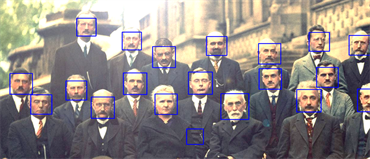Figure 10. Face detection results and test results

Study on Convolutional Neural Networks Cascade for Real-time Face Recognition Methods[J]. 计算机科学与应用, 2020, 10(01): 11-20. https://doi.org/10.12677/CSA.2020.101002

1. 1. 黄明祥, 林咏章. 信息与网络安全概论[M]. 第3版. 北京: 清华大学出版社, 2010.

2. 2. LeCun, Y., Boser, B., Denker, J.S., Henderson, D., Howard, R.E., Hubbard, W. and Jackel, L.D. (1989) Backpropagation Applied to Hand-written Zip Code Recognition. Neural Computation, 1, 541-551. https://doi.org/10.1162/neco.1989.1.4.541

3. 3. LeCun, Y., Bottou, L., Bengio, Y. and Haffner, P. (1998) Gradi-ent-Based Learning Applied to Document Recognition. Proceedings of the IEEE, 86, 2278-2324. https://doi.org/10.1109/5.726791

4. 4. Sutskever, K.I. and Hinton, G. (2012) ImageNet Classification with Deep Convolutional Neural Networks. Advances in Neural Information Processing Systems, 25, 1106-1114.

5. 5. Szegedy, C., Liu, W., Jia, Y.Q., Sermanet, P., Reed, S.E., Anguelov, D., Erhan, D., Vanhoucke, V. and Rabinovich, A. (2015) Going Deeper with Convolutions. 2015 IEEE Conference on Computer Vision and Pattern Recognition, Boston, MA, 7-12 June 2015, 1-9. https://doi.org/10.1109/CVPR.2015.7298594

6. 6. Simonyan, K. and Zisserman, A. (2014) Very Deep Convolutional Networks for Large-Scale Image Recognition. Computer Science, 1-14.

7. 7. He, K.M., Zhang, X.Y., Ren, S.Q. and Sun, J. (2016) Deep Residual Learning for Image Recognition. 2016 IEEE Conference on Computer Vision and Pattern Recognition (CVPR), Las Vegas, NV, 27-30 June 2016, 770-778.

8. 8. Fukushima, K. (1980) Neo-cognitron: A Self-Organizing Neural Network Model for Amechanism of Pattern Recognition Unaffected by Shift in Po-sition. Biological Cybernetics, 36, 93-202. https://doi.org/10.1007/BF00344251

9. 9. Oeschoten, M.A., Kenemans, J.L., van Engeland, H. and Kemner, C. (2007) Face Processing in Pervasive Developmental Disorder (PDD): The Roles of Expertise and Spatial Frequency. Journal of Neural Transmission, 114, 1619-1629. https://doi.org/10.1007/s00702-007-0780-y

10. 10. Calder, A.J., Young, A.W., Keane, J. and Dean, M. (2000) Con-figural Information in Facial Expression Perception. The Journal of Experimental Psychology: Human Perception and Performance, 26, 527-551.

11. 11. Casco, C., Campana, G, Grieco, A. and Fuggetta, G. (2004) Perceptual Learning Mod-ulates Electrophysiological and Psychophysical Response to Visual Texture Segmentation in Humans. Neuroscience Let-ters, 371, 18-23. https://doi.org/10.1016/j.neulet.2004.08.005

12. 12. Dakin, S.C., Hess, R.F., Ledgeway, T. and Achtman, R.L. (2002) What Causes Nonmonotonic Tuning of fMRI Response to Noisy Images? Current Biology, 12, R476-R477. https://doi.org/10.1016/S0960-9822(02)00960-0

13. 13. Deruelle, C., Rondan, C., Gepner, B. and Tardif, C. (2004) Spatial Frequency and Face Processing in Children with Autism and Asperger Syndrome. Journal of Autism and Devel-opmental Disorders, 34, 199-210. https://doi.org/10.1023/B:JADD.0000022610.09668.4c

14. 14. Deruelle, C., Rondan, C., Salle-Collemiche, X., Bas-tard-Rosset, D. and Da Fonséca, D. (2008) Attention to Low- and High-Spatial Frequencies in Categorizing Facial Iden-tities, Emotions and Gender in Children with Autism. Brain and Cognition, 66, 115-123. https://doi.org/10.1016/j.bandc.2007.06.001

15. 15. Eimer, M. (2000) The Face-Specific N170 Component Reflects Late Stages in the Structural Encoding of Faces. NeuroReport, 11, 2319-2324. https://doi.org/10.1097/00001756-200007140-00050

16. 16. Ellemberg, D., Lewis, T.L., Maurer, D., Lui, C.H. and Brent, H.P. (1999) Spatial and Temporal Vision in Patients Treated for Bilateral Congenital Cataracts. Vision Research, 39, 3480-3489. https://doi.org/10.1016/S0042-6989(99)00078-4